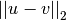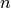# scipy.spatial.distance.euclidean¶

scipy.spatial.distance.euclidean(u, v)

Computes the Euclidean distance between two n-vectors u and v, which is defined asParameters : u : ndarray An-dimensional vector. v : ndarray An-dimensional vector. d : double The Euclidean distance between vectors u and v.

#### Previous topic

scipy.spatial.distance.dice

#### Next topic

scipy.spatial.distance.hamming